Search IntMath
Close

450+ Math Lessons written by Math Professors and Teachers

5 Million+ Students Helped Each Year

1200+ Articles Written by Math Educators and Enthusiasts

Simplifying and Teaching Math for Over 23 Years

# Maximum value of a Poisson Distribution

By Murray Bourne, 19 Apr 2010

When I was going through Probability Theory in Interactive Mathematics, I found Poisson Distribution quite interesting.

When I tried to find the maximum Probability using differentiation I suddenly realized that I don't know how to deal with differentiation when there is the Factorial in formula. Internet search gave me quite complicated solutions so I left it behind for now. If you find this problem interesting then it can be an interesting topic for the newsletter.

Well, I agree it's an interesting problem, so here we go. First, some background on what Jegor is asking.

## Poisson Distribution - an Overview

Whenever there is a court case involving rare diseases (like Parkinson's Disease (which I wrote about in Michael J. Fox and the Parkinson’s cluster) or cancer (Probability of a cancer cluster: 1 in a million), the prosecution and defense argue over their interpretations of the Poisson Distribution. [See the whole thing here: Poisson Distribution.]

Poisson Distribution only applies if the events in question are independent. We also need to count the number of "successes" (or failures), so the variables involved need to be non-negative integers.

The formula for the probability distribution of a Poisson random variable X is given by the formula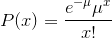where

x is a non-negative integer (That is, 0, 1, 2, 3, ...)

e = 2.71828... (the well-known constant)

μ = mean number of successes in the given time interval or region of space

Typically, this will produce a distribution as follows.

Let's take Example 2 from my Poisson Distribution page. In that example, we had a table indicating the number of flaws found in a manufacturing process and we needed to determine the probabilities of finding flaws in future manufacturing runs.

The average (mean) was μ = 2.3 and after applying the above formula for x = 0, x = 1, x = 2, etc, we obtained this histogram (bar chart, not a continuous curve) for the probability.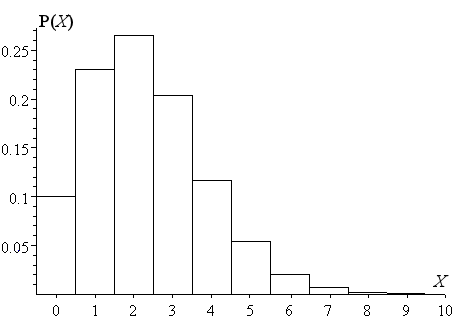## Poisson Distribution for Continuous Variables

By definition, the x in our Poisson formula is discrete, since we need to count the number of flaws (or sickness cases, or whatever).

So we can't actually find the derivative of the Poisson Distribution, since differentiation only works for continuous functions. Also, x! (factorial x) only has meaning if x is a non-negative integer.

However, as a hypothetical exercise, it could be interesting to proceed.

In an earlier article, I wrote about how the Gamma Function gives us continuous values for x!. That is, we can find some meaning for expressions like 3.5!.

Now, to find the derivative of the formula,we need to be able to find the derivative of x! (that is, "x factorial").

Recall the following Gamma Function, which is analogous to x!: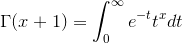The derivative of the Gamma Function is defined as: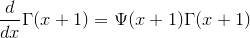And what is that Psi (Ψ)function in there? It is defined as the "logarithmic derivative of the gamma function"! This is rather a circular definition, and not very helpful, so let's see what we can figure out using graphs.

## The Graph of the Poisson Function

Let's continue to assume we have a continuous variable x and graph the Poisson Distribution,It will be a continuous curve, as follows.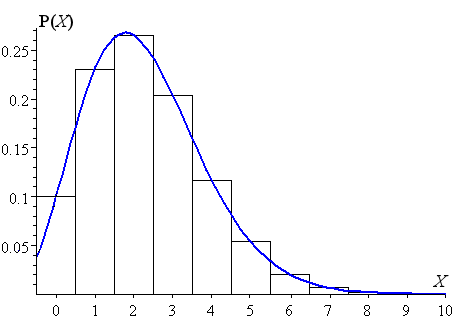I have included the (valid) discrete case (the black rectangles) for comparison. (You can graph this yourself using Wolfram | Alpha. You can enter it as follows (e^-2.3)(2.3^x)/x! from 0 to 10.)

Our aim is to find the value of x that gives maximum value of the probability P(X). We can see it is slightly less than x = 2.

We can graph the derivative of the Poisson distribution using a computer algebra system, as follows. The value we need will be given by the X-axis intercept.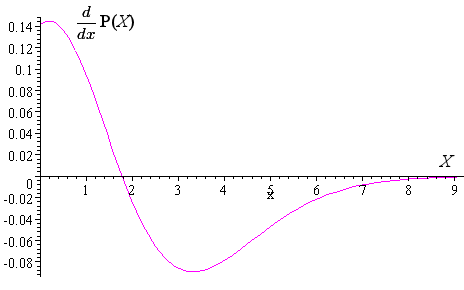(You can also get Wolfram \ Alpha to do this for you:

Zooming in on the portion near x = 2, we obtain: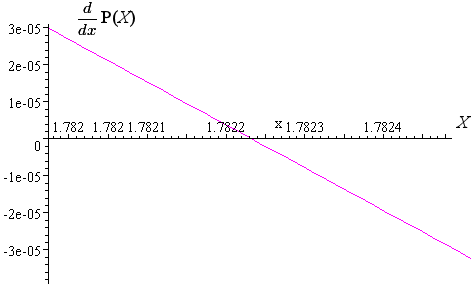From the above, we can see the maximum will occur when x = 1.78223.

Substituting this value into the Poisson expression gives us the maximum value: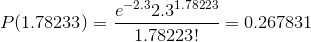## Conclusion

Our result tallies nicely with the graph of the "continuous" Poisson Distribution above. That is, we have successfully found the "maximum". We did it using graphical means, since an algebraic approach appears rather obscure.

However, while we have found a hypothetical "maximum" value of the Poisson Distribution for this example, it is not valid since Poisson Distribution only applies for discrete, countable variables.

### 9 Comments on “Maximum value of a Poisson Distribution”

1. Doug Gerrard says:

On the maximum of the Poisson Distribution: Recognizing that the distribution is discrete and therefore is not subject to continuous function rules, we can still utilize the fact that the maximum frequency occurs at the "Mode", which differs only slightly from the Median (slightly to the left of the Mean, Mu = 2.3, for a right-skewed distribution). In other words, the mean value, 2.3, gets us within one unit of the x-value we seek. Calculating adjacent frequencies confirms our answer.

2. Murray says:

Hi Doug and thanks for your input.

Yes, I realize the above process is overkill, but that's what the reader was trying to do!

3. Damon says:

It’s funny; as soon as I see the words “Poisson Distribution” I tend to immediately think discrete units and shut off all thought of differentiation or the use of the properties of continuous functions. With a mean of 2.3, we know that the most likely discrete outcome will be either 2 or 3. After you plug in each value, you find that x=2 results in the greater probability.

Thus, I would have answered that the greatest probability of 26.52% occurs when x=2 (or there are 2 occurrences) during a given time interval (or space). I would have been perfectly satisfied with this answer, but I would have missed out on an insight into an alternate line of thinking.

I found this article to be a nice theoretical exercise with an interesting result that hopefully will help me to think outside of the box more often. To me this was not overkill; it was enjoyable throughout. Please keep it up.

4. Waseem says:

The above solution is very nice and insightful and permits us to get out of the traditional thinking box. Thanks Murray for the pain.

An algebraic approach is to simplify the ratio between successive terms: P(x+1)/P(x) = μ/(x+1).

If the maximum occurs at a given value of x, then
P(x+1)/P(x) < 1 and P(x)/P(x-1) > 1,
which jointly imply that x <= μ <= x+1.

6. bushra says:

is a poisson distribution an inreasing or a decreasing function ????

7. shah kartik says:

see,i have a doubt.if mean is so close to mode ....i mean if it is 3then mode are 3 and 2 and if mean is 2.3 it is 2 so shouldn't the possion be more of symmetric?

8. Avinash Sahay says:

If lambda is an integer, then maximum is at lambda and lambda - 1
Otherwise maximum is at floor(lambda)
This can be proved without any approximation. See the following video

9. Upendra Joshi says:

Avinash Sahay, that is a nice, simple explaination.

### Comment Preview

HTML: You can use simple tags like <b>, <a href="...">, etc.

To enter math, you can can either:

1. Use simple calculator-like input in the following format (surround your math in backticks, or qq on tablet or phone):
a^2 = sqrt(b^2 + c^2)
(See more on ASCIIMath syntax); or
2. Use simple LaTeX in the following format. Surround your math with $$ and $$.
$$\int g dx = \sqrt{\frac{a}{b}}$$
(This is standard simple LaTeX.)

NOTE: You can mix both types of math entry in your comment.